Discrete Mathematics and Combinatorics Commons™

945 Full-Text Articles 1,167 Authors 104,022 Downloads95 Institutions

All Articles in Discrete Mathematics and Combinatorics

945 full-text articles. Page 31 of 35.

Combinatorics Using Computational Methods, 2012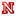University of Nebraska-Lincoln

Combinatorics Using Computational Methods, Derrick Stolee

Dissertations, Theses, and Student Research Papers in Mathematics

Computational combinatorics involves combining pure mathematics, algorithms, and computational resources to solve problems in pure combinatorics. This thesis provides a theoretical framework for combinatorial search, which is then applied to several problems in combinatorics. Some results in space-bounded computational complexity are also presented.

The 1, 2-Conjecture For Graphs With Relatively Small Chromatic Number, 2012University of Illinois at Urbana–Champaign

The 1, 2-Conjecture For Graphs With Relatively Small Chromatic Number, Sogol Jahanbekam, Douglas West

Faculty Publications

No abstract provided.

Exploring The On-Line Partitioning Of Posets Problem, 2012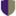Scripps College

Exploring The On-Line Partitioning Of Posets Problem, Leah F. Rosenbaum

Scripps Senior Theses

One question relating to partially ordered sets (posets) is that of partitioning or dividing the poset's elements into the fewest number of chains that span the poset. In 1950, Dilworth established that the width of the poset - the size of the largest set composed only of incomparable elements - is the minimum number of chains needed to partition that poset. Such a bound in on-line partitioning has been harder to establish, and work has evalutated classes of posets based on their width. This paper reviews the theorems that established val(2)=5 and illustrates them with examples. It also covers ...

2012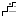Illinois Mathematics and Science Academy

Session D-3: Discrete Mathematics: A Great Curriculum Connector, Donald Porzio

Professional Learning Day

Many topics that fall under the umbrella of Discrete Mathematics cut across the traditional high school curriculum areas of algebra, geometry, and pre-calculus. Come try some classroom-ready hands-on Discrete Mathematics activities that illustrate the true interconnectedness of mathematics.

Fixed Points And Excedances In Restricted Permutations, 2012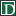Dartmouth College

Fixed Points And Excedances In Restricted Permutations, Sergi Elizalde

Open Dartmouth: Faculty Open Access Scholarship

Using an unprecedented technique involving diagonals of non-rational generating functions, we prove that among the permutations of length \$n\$ with \$i\$ fixed points and \$j\$ excedances, the number of 321-avoiding ones equals the number of 132-avoiding ones, for any given \$i,j\$. Our theorem generalizes a result of Robertson, Saracino and Zeilberger. Even though bijective proofs have later been found by the author jointly with Pak and with Deutsch, this paper contains the original analytic proof that was presented at FPSAC 2003.

Introduction Aux Méthodes D’Intégrale De Chemin Et Applications, 2012SelectedWorks

Introduction Aux Méthodes D’Intégrale De Chemin Et Applications, Nour-Eddiine Fahssi

Nour-Eddine Fahssi

These lecture notes are based on a master course given at University Hassan II - Agdal in spring 2012.

2012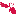Linfield College

Complete Multipartite Graphs And The Relaxed Coloring Game, Charles Dunn

Faculty Publications

Let k be a positive integer, d be a nonnegative integer, and G be a finite graph. Two players, Alice and Bob, play a game on G by coloring the uncolored vertices with colors from a set X of k colors. At all times, the subgraph induced by a color class must have maximum degree at most d. Alice wins the game if all vertices are eventually colored; otherwise, Bob wins. The least k such that Alice has a winning strategy is called the d-relaxed game chromatic number of G, denoted χ gd (G). It is known that ...

The Minimum Of The Maximum Rectilinear Crossing Numbers Of Small Cubic Graphs, 2012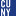Harvard University

The Minimum Of The Maximum Rectilinear Crossing Numbers Of Small Cubic Graphs, Matthew Alpert, Jens-P. Bode, Elie Feder, Heiko Harborth

Publications and Research

Here we consider the minimum of the maximum rectilin­ear crossing numbers for all d-regular graphs of order n. The case of connected graphs only is investigated also. For d = 3 exact values are determined for n are less than or equal to 12 and some estimations are given in general.

Finite Factors Of Bernoulli Schemes And Distinguishing Labelings Of Directed Graphs, 2012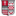Sacred Heart University

Finite Factors Of Bernoulli Schemes And Distinguishing Labelings Of Directed Graphs, Andrew Lazowski, Stephen M. Shea

Mathematics Faculty Publications

A labeling of a graph is a function from the vertices of the graph to some finite set. In 1996, Albertson and Collins defined distinguishing labelings of undirected graphs. Their definition easily extends to directed graphs. Let G be a directed graph associated to the k -block presentation of a Bernoulli scheme X . We determine the automorphism group of G , and thus the distinguishing labelings of G . A labeling of G defines a finite factor of X . We define demarcating labelings and prove that demarcating labelings define finitarily Markovian finite factors of X . We use the Bell numbers to find ...

Stochastic Processes Induced By Singular Operators, 2012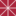Chapman University

Stochastic Processes Induced By Singular Operators, Daniel Alpay, Palle Jorgensen

Mathematics, Physics, and Computer Science Faculty Articles and Research

In this paper we study a general family of multivariable Gaussian stochastic processes. Each process is prescribed by a fixed Borel measure σ on Rn. The case when σ is assumed absolutely continuous with respect to Lebesgue measure was stud- ied earlier in the literature, when n = 1. Our focus here is on showing how different equivalence classes (defined from relative absolute continuity for pairs of measures) translate into concrete spectral decompositions of the corresponding stochastic processes under study. The measures σ we consider are typically purely singular. Our proofs rely on the theory of (singular) unbounded operators in Hilbert ...

New Topological C-Algebras With Applications In Linear Systems Theory, 2012Chapman University

New Topological C-Algebras With Applications In Linear Systems Theory, Daniel Alpay, Guy Salomon

Mathematics, Physics, and Computer Science Faculty Articles and Research

Motivated by the Schwartz space of tempered distributions S′ and the Kondratiev space of stochastic distributions S−1 we define a wide family of nuclear spaces which are increasing unions of (duals of) Hilbert spaces H′p,p∈N, with decreasing norms |⋅|p. The elements of these spaces are functions on a free commutative monoid. We characterize those rings in this family which satisfy an inequality of the form |f∗g|p≤A(p−q)|f|q|g|p for all p≥q+d, where * denotes the convolution in the monoid, A(p−q) is a strictly positive number and ...

White Noise Based Stochastic Calculus Associated With A Class Of Gaussian Processes, 2012Chapman University

White Noise Based Stochastic Calculus Associated With A Class Of Gaussian Processes, Daniel Alpay, Haim Attia, David Levanony

Mathematics, Physics, and Computer Science Faculty Articles and Research

Using the white noise space setting, we define and study stochastic integrals with respect to a class of stationary increment Gaussian processes. We focus mainly on continuous functions with values in the Kondratiev space of stochastic distributions, where use is made of the topology of nuclear spaces. We also prove an associated Ito formula.

An Interpolation Problem For Functions With Values In A Commutative Ring, 2012Chapman University

An Interpolation Problem For Functions With Values In A Commutative Ring, Daniel Alpay, Haim Attia

Mathematics, Physics, and Computer Science Faculty Articles and Research

It was recently shown that the theory of linear stochastic systems can be viewed as a particular case of the theory of linear systems on a certain commutative ring of power series in a countable number of variables. In the present work we study an interpolation problem in this setting. A key tool is the principle of permanence of algebraic identities.

Schur Functions And Their Realizations In The Slice Hyperholomorphic Setting, 2012Chapman University

Schur Functions And Their Realizations In The Slice Hyperholomorphic Setting, Daniel Alpay, Fabrizio Colombo, Irene Sabadini

Mathematics, Physics, and Computer Science Faculty Articles and Research

In this paper we start the study of Schur analysis in the quaternionic setting using the theory of slice hyperholomorphic functions. The novelty of our approach is that slice hyperholomorphic functions allows to write realizations in terms of a suitable resolvent, the so called S-resolvent operator and to extend several results that hold in the complex case to the quaternionic case. We discuss reproducing kernels, positive definite functions in this setting and we show how they can be obtained in our setting using the extension operator and the slice regular product. We define Schur multipliers, and find their co-isometric realization ...

On The Class Rsi Of J-Contractive Functions Intertwining Solutions Of Linear Differential Equations, 2012Chapman University

On The Class Rsi Of J-Contractive Functions Intertwining Solutions Of Linear Differential Equations, Daniel Alpay, Andrey Melnikov, Victor Vinnikov

Mathematics, Physics, and Computer Science Faculty Articles and Research

In this paper we extend and solve in the class of functions RSI mentioned in the title, a number of problems originally set for the class RS of rational functions contractive in the open right-half plane, and unitary on the imaginary line with respect to some preassigned self-adjoint matrix. The problems we consider include the Schur algorithm, the partial realization problem and the Nevanlinna-Pick interpolation problem. The arguments rely on the one-to-one correspondence between elements in a given subclass of RSI and elements in RS. Another important tool in the arguments is a new result pertaining to the classical tangential ...

Integrated Methods For Optimization, 2nd Ed, 2011Carnegie Mellon University

Integrated Methods For Optimization, 2nd Ed, John Hooker

John Hooker

No abstract provided.

2011Carnegie Mellon University

Toward Unification Of Exact And Heuristic Optimization Methods, John Hooker

John Hooker

No abstract provided.

Highly Connected Multicoloured Subgraphs Of Multicoloured Graphs, 2011Illinois Mathematics and Science Academy

Highly Connected Multicoloured Subgraphs Of Multicoloured Graphs, H. Liu, R. Morris, N. Prince

Hua Kun Liu

Suppose the edges of the complete graph on n vertices, E(Kn), are coloured using r colours; how large a k-connected subgraph are we guaranteed to find, which uses only at most s of the colours? This question is due to Bollobás, and the case s=1 was considered in Liu et al. [Highly connected monochromatic subgraphs of multicoloured graphs, J. Graph Theory, to appear]. Here we shall consider the case s is greater than or equal to 2, proving in particular that when s=2 and r+1 is a power of 2 then the answer lies between 4n ...

Combinatorics Of Two-Toned Tilings, 2011Harvey Mudd College

Combinatorics Of Two-Toned Tilings, Arthur T. Benjamin, Phyllis Chinn, Jacob N. Scott '11, Greg Simay

All HMC Faculty Publications and Research

We introduce the function a(r, n) which counts tilings of length n + r that utilize white tiles (whose lengths can vary between 1 and n) and r identical red squares. These tilings are called two-toned tilings. We provide combinatorial proofs of several identities satisfied by a(r, n) and its generalizations, including one that produces kth order Fibonacci numbers. Applications to integer partitions are also provided.

2011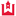Western Kentucky University

Cagan Type Rational Expectations Model On Time Scales With Their Applications To Economics, Funda Ekiz

Masters Theses & Specialist Projects

Rational expectations provide people or economic agents making future decision with available information and past experiences. The first approach to the idea of rational expectations was given approximately fifty years ago by John F. Muth. Many models in economics have been studied using the rational expectations idea. The most familiar one among them is the rational expectations version of the Cagans hyperination model where the expectation for tomorrow is formed using all the information available today. This model was reinterpreted by Thomas J. Sargent and Neil Wallace in 1973. After that time, many solution techniques were suggested to solve the ...